# Matching Supply with Demand

## Quiz 9 : Quality Management, Statistical Process Control, and Six Sigma CapacityLooking for Management Homework Help?

## Quiz 9 :Quality Management, Statistical Process Control, and Six Sigma Capacity

Question Type(Quality) Consider the following potential quality problems: • Wine that is served in a restaurant sometimes is served too warm, while at other times it is served too cold. • A surgeon in a hospital follows the hygiene procedures in place on most days, but not all days. • A passenger traveling with an airline might be seated at a seat with a defective audio system. • An underwriter in a bank might sometimes accidentally approve loans to consumers that are not creditworthy. For each of these potential problems: a. What type of data would you collect b. What type of control charts would you use
Free
Essay

The type of data that needs to be collected depends on the situation and the problem in hand. Often for defect or defective parts count, the data will be attribute in nature. For other types of situation, the data needs to be in variable form when the outcome can be measured. For control chart, the choice should depend on the what type of output the user wants to monitor. For example, an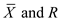chart will be used for an output for which the criteria are to monitor or control a measurable parameter or a ratio data. For discrete data,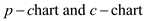are used for proportion of defectives and defect counts respectively.
a.
Note the following table explain the choice of data for each of the four situations.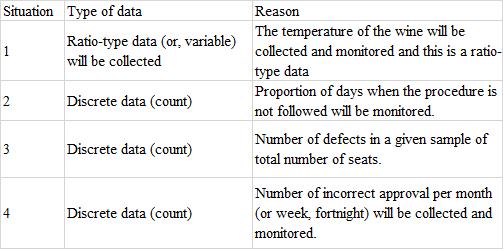b.
Note the following table explain the choice of control chart for each of the four situations.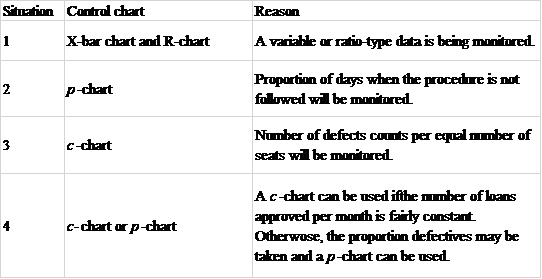Tags(Process with Rework) Consider the following three-stage production process of glass ceramics, which is operated as a worker-paced line.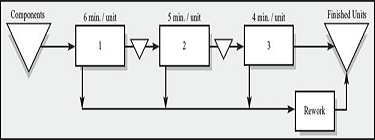The process is experiencing severe quality problems related to insufficiently trained workers. Specifically, 20 percent of the parts going through operation 1 are badly processed by the operator. Rather than scrapping the unit, it is moved to a highly skilled rework operator, who can correct the mistake and finish up the unit completely within 15 minutes. The same problem occurs at station 2, where 10 percent of the parts are badly processed, requiring 10 minutes of rework. Station 3 also has a 10 percent ratio of badly processed parts, each of them requiring 5 minutes by the rework operator. a. What is the utilization of statioN2 if work is released into the process at a rate of 5 units per hour b. Where in the process is the bottleneck Why (Remember, the bottleneck is the resource with the lowest capacity, independent of demand.) c. What is the process capacity
Free
Essay

When a portion of output from a process step is reworked, the overall capacity of the step reduces. The reduction of capacity of a bottleneck resource causes the reduction of the overall process capacity. For an unlimited demand scenario, this will result in lower throughput.
a.
Work is being released at a rate of 5 units per hour.
Operation 1
Processing time is 6 minutes per unit.
Rework rate is 20%
Rework processing time per unit is 15 minutes.
So, out of the 5 units, number of reworks generated =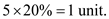So,So,OperatioN2
Processing time is 5 minutes per unit.
Rework rate is 10%
Rework processing time per unit is 10 minutes
So, out of the 5 units, number of reworks generated =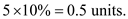So,So,Operation 3
Processing time is 4 minutes per unit.
Rework rate is 10%
Rework processing time per unit is 5 minutes
So, out of the 5 units, number of reworks generated =So,So,Note that all the three operations are having capacity more than the release rate. So, the release rate of 5 units per hour will be the flow rate.So, the utilization operatioN2 is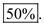b.
The lowest capacity step is Operation 1 with capacity of 6.67 units per hour.
So, the bottleneck is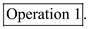c.
The processing capacity is the minimum of demand (i.e. the release rate) and capacity of the bottleneck (i.e. operation 1).
So,So, the present capacity of the process is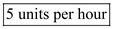. If the release rate is not a constraint and can be increased as required, the process capacity will be the capacity of the bottleneck i.e.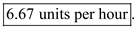Tags